Courses

# NCERT Solution, Area and its Boundary, class 5, Mathematics Class 5 Notes | EduRev

## Class 5 : NCERT Solution, Area and its Boundary, class 5, Mathematics Class 5 Notes | EduRev

The document NCERT Solution, Area and its Boundary, class 5, Mathematics Class 5 Notes | EduRev is a part of the Class 5 Course Mathematics for Class 5 (V) - CBSE and NCERT Curriculum.
All you need of Class 5 at this link: Class 5

CERT Solution, Area and its Boundary, class 5, Mathematics

1. (a) Arbaz plans to tile his kitchen floor with green square tiles. Each side of the tile is 10 cm. His kitchen is 220 cm in length and 180 cm wide. How many tiles will he need?

Ans.

(a) We know that,

Area of a rectangle = Length x Breadth

Area of the floor of kitchen = (Length x Breadth)

= (220 x 180) square cm.

Also, we know that

Area of a square = Side x Side

Area of one tile = (10 x10) square cm

Number of tiles required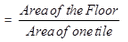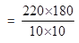= 22 x 18 = 396

(b) The fencing of a square garden is 20 m in length. How long is one side of the garden?

Ans.

(b) We have, Perimeter = 20 m

Side =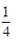x Perimeter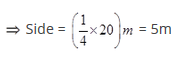(c) A thin wire 20 centimetres long is formed into a rectangle. If the width of this rectangle is 4 centimetres, what is its length?

Ans.

(c) We have, Perimeter = 20 cm and Breadth = 4cm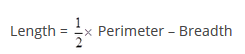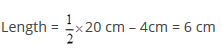= 10 cm – 4 cm = 6 cm

(d) A square carom board has a perimeter of 320 cm. How much is its area?

Ans. (d) We have, Perimeter = 320 cm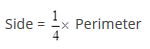Side = (1/4) x 320 cm = 80 cm

Area = Side x Side

= (80 x 80) square cm = 6400 square cm

(e) How many tiles like the triangle given here will fit in the white design?

Area of design = …………………. Square cm

Ans. (e) Area of the design = 1 full square + 4 half square

= (1 + 2) full square

= 3 full squares

= 3 x 1 square cm = 3 square cm

Area of one tile = half of the cm square

=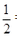square cm

Number of tiles =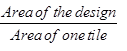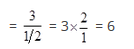(f) Sanya, Aarushi, Manav and Kabir made greeting cards. Complete the table for their cards: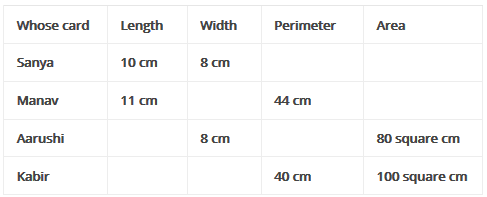Ans.

(f)

In case of Sanya:

We have Length = 10 cm, Width = 8 cm

Perimeter = 2 (Length + Width)

= 2 (10+8) cm

= 2 x 18 cm = 36 cm

Area = Length x Width

= 10 x 8 square cm

In Case of Manav:

We have, Length = 11 cm, Perimeter = 44 cm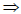2 (Length + Width) = 44 cmLength + Width = 22 cm11 cm + Width = 22 cm

Width = 22 cm- 11 cm = 11 cm

And, Area = Length x Width

= 11 x 11 square cm

= 121 square cm

In Case of Aarushi:

We have, Width =8 cm

Area = 80 square cmLength x Width = 80 square cmLength x 8 cm = 80 square cm

Length = 80/8 cm = 10 cm

And, Perimeter = 2(Length + Width)

= 2(10 +8) cm

= 2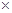18 cm = 36 cm

In Case of Kabir:

We have, Perimeter = 40 cm

Area = 100 square cm2 (Length+ Width) = 40 cm

And, Length +Width = Length and Width

i.e. Length +Width = 20Width = 20 – Length ……..(1)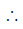Length x Width = 100Length (20 – length)20 (length) – (length)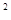= 100Length– 20 Length +100 = 0(Length – 10)= 0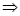Length-10 = 0Length = 10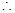Width = 20 – Length = 20 -10 = 10

i.e. Length = 10 cm and Width = 10 cm

Therefore, the complete table is as under: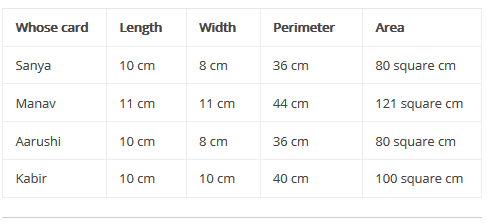2. Take a thick paper sheet of length 14 cm and width 9 cm. You can also use an old postcard.

(a) What is its area? What is its perimeter?

Ans.

(a) We know that,

Area of a rectangle = Length x Breadth

Area of a thick paper sheet = (Length x Breadth)

= (14x9) square cm = 126 square cm

Perimeter of a rectangular sheet

= 2(14 + 9) cm

= 2 x 23 cm = 46cm

(b) Now cut strips of equal sizes out of it.

Ans.

(b) Let us cut strips of equal sizes out of the given paper sheet of length 14 cm and width  9 cm.

(c) How long it your belt?

Ans.

(c) Strips having width 1 cm:

There will be 9 strips of width 1 cm and length 14 cm. Let us join these strips end to end using tape to make a belt.

Its length
= (14+14+14+14+14+14+14+14+14)

= 9 x 14 cm = 126 cm

It’s perimeter = 2(126+1) cm = 2 x 127 cm = 254 cm

(d) What is its Perimeter?

Ans. (d) Strips having length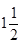cm:

There will be 6 strips of widthcm and length 14 cm. Let us join these strips end to end using tape to make a belt.

It’s length = (14+14+14+14+14+14) cm

= 614 cm = 84 cm

It’s perimeter = 2 (84 + 1.5) cm = 2 x 85 – 5 cm = 171 cm

(e) Whose belt is the longest in the class?

Ans. (e) Strips having width 3 cm:

There will be 3 strips of width 3 cm and length 14 cm.

Let us join these strips end to end using tape to make a belt.

It’s length = (14+14+14) cm = 3 x 14 cm = 42 cm

It’s perimeter = 2(42+3) cm = 2 x 45 cm = 90 cm

Clearly, the belt with shortest width is the longest one. Thus, the belt having width 1 cm is the longest in the class. So, my belt is the longest.

3. Let’s Discuss:

(a) Why did some of your friends get longer belt than others?

Ans.

(a) Some of my friends gets longer belts than others as the width of their belts are shorter than that of the other’s belt.

(b) Is the area of your belt as the same as the area of the postcard? Why or why not?

Ans.

(b) The area of the belt is the same as the area of the postcard because it is made of the postcard without wastage.

(c) What will you do get the longer belt next time?

Ans.

(c) I will get the longer belt next time by ensuring that the width of my belt should be shorter than other’s belt.

4. People People Everywhere:

(A) You can play this game in a ground.

Make two squares of one square metre each.

(a) How many of you can sit in one square metre?

Ans.

(a)Three of us can sit in one square metre.

(b) How many of you can stand in it?

Ans.

(b) Four of us can stand in it.

(c) Which team could make children stand in their square? How many?

Ans.

(c) Team A could make more children to stand in their square. They are five in number.

(d) Which team could make more children sit in their square? How many?

Ans.

(d) Team A could make more children to sit in their square. They are four in number.

(B) Measure the length of the floor of your children in metres. Also measure the width.

(a) What is the area of the floor of your children in square metres?

Ans.

(a)Let’s measure the floor of the classroom in metres.

We find that its length = 10 metres and width = 6 metres

It’s area = (Length x Breadth)

= (10 x 6) square metres

= 60 square metres.

(b) How many children are there in your class?

Ans.

(b) There are 60 children in my class.

(c) So how many children can sit in one square metre?

Ans.

(c) Number of children who can sit in one square metre =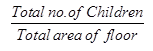= 60/60 =1

Thus, one child can sit in one square metre.

(d) If you want to move around easily then how many children do you think should be there in one square metre?

Ans.

(d) To move around easily, I think 2 children may sit in 3 square metres space.

5. Share the Land:

Nasreena is a farmer who want to divide her land equally among her three children, Chumki, Jhumri and Imran. She wants to divide the land so that each piece of land has one tree. Her land looks like this.

(a) Can you divide the land equally? Show how you will divide it. Remember each person has to get a tree. Colour each person’s piece of land differently.

Ans.

(a) Yes, I can divide the land equally among Nasreena’s three children. Chumki, Jhumri and Imran such that each gets one tree. Each person’s piece of land is coloured differently as shown in the figure.

(b) If each square on this page is equal to 1 square metre of land, how much land will each of her children get?  … sq. m

Ans.

(b) Let Chumki’s land be ABCDEF, Jhumri’s land be BGHIJC and that of Imran be DJIHKE.

Total land in area = 10 x 9 m = 90 square metres

Since each person gets equal land.

Each share = 90/3 square metres = 30 square metres

Thus, each chold gets 30 square metres of land.

(c) Chumki Jumhri and Imran need wire to make a fence.

Ans.

(c) For Fencing:

Perimeter of Chumki’s land ABCDEF = AB + BC + CD + DE + EF + FA

= (4 + 6 +2 + 3+ 2+ 9) m = 26 m

Perimeter of Jhumri’s land BGHIJC = BG + GH + HI + IJ + JC + CB

= (6 + 4+ 3 + 2 + 3+ 6) m = 24 m

Perimeter of Imran’s land DJIHKE = DJ + JI + IH + HK + KE + ED

= (5 + 2 + 3 + 5 + 8 +3) m = 26 m

(d) Who will need the longest wire for fencing?

Ans.

(d) Clearly, Chumki and Imran needs longest but equal wires to make a fence.

(e) How much wire in all will the three need?

Ans.

(e) Total wire needed = (26 + 24 + 26) m = 76 m

(a) The perimeter of square A is .. cm.

Ans.

(a) The perimeter of square A is 4 x 3 cm = 12 cm.

(b) The side of square B is .. cm.

Ans.

(b) The side of square B is 2 x 3 cm = 6 cm.

(c) The area of square B is .. square cm.

Ans.

(c) The area of square B is 6 cm x 6 cm = 36 sq. cm.

(d) The area of square B is .. the times the area of square A.

Ans.

(d) The area of sq. B is four times of the area of square A.

(e) The perimeter of square B is  .. cm.

Ans.

(e) The perimeter of square B is 4 x 6 cm = 24 cm.

(f) The perimeter of square B is .. times the perimeter of square A.

Ans.

(f) The perimeter of square B is two times the perimeter of square A.

(A) Which shape has the biggest area? How much?

Ans. (A) To find the area of shape A

Figure A contains 9 complete squares, 2 half squares, 7 more than half squares and 8 less than half squares. Neglecting less than half squares and consider more than half square as complete squares. Therefore, the approximate area of figure A.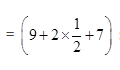square cm = (9+1+7) square cm = 17 square cm

Similarly, the area of shape B will be approximately equal to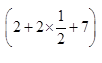square cm = 10 square cm.

And, the area of shape Csquare cm

=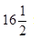sq.cm

Clearly, the shape A has the biggest area.

It is (17 – 10) i.e.  7 sq.cm more that of shape B and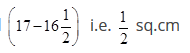more than that of the slope.

(B) Which shape has the smallest area? How much? What is the perimeter of the shape?

Also make a triangle, a square, a rectangle and a circle. Find which shape has the biggest area and which has the smallest?

Ans.

(B) The shape B has the smallest area.

It is (17-10) i.e. sq. cm less than of shape A and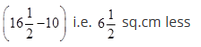than that of shape C.

The perimeter of this shape is 15 cm.

Let us also make a triangle, a square, a rectangle and circle using the same 15 cm thread.

Area of the triangle =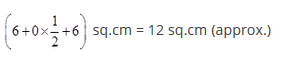Area of square =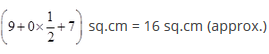Area of the triangle =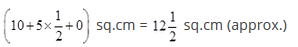Area of the circle =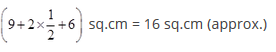The circle and the square have the biggest area and the triangle has the smallest area.

8. Save the Birds:

There are two beautiful lakes near a village. People come for boating and picnics in both the lakes. The village Panchayat is worried that with the noise of the boats the birds will stop coming. The Panchayat wants motor boats in only one lake. The other lake will be saved for the birds to make their nests.

(a) How many cm in the length of the boundary of lake A in the drawing?

Ans.

(a) The length of the boundary of lake A in the drawing is 33 cm.

(b) What is the length of the boundary of lake B in the drawing?

Ans.

(b) The length of the boundary of lake B in the drawing is 26 cm.

(c) A longer boundary around the lake will help more birds to lay their eggs. So which lake should be kept for birds? Which lake should be used for boats?

Ans.

(c) Since 1 cm on drawing = 1 km on the ground33 cm represents 33 x 1 km i.e. 33 km.

(d) Find the area of lake B on the drawing in square cm. What is the actual area in square km?

Ans.

(d) Lake B contains 15 complete squares, 3 half squares, 8 more than half squares and 2 less than half squares. Neglecting less than half squares and considering more than half squares the approximate area of lake B on drawing.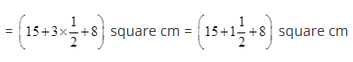Actual area of lake B =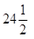square km

9. King’s Story:

The king was very happy with carpenters Chaggu and Anar. They had made a very big and beautiful bed for him. So as gifts the king wanted to give some land to Chaggu, and some gold to Anar.

Chaggu was happy. He took 100 metres of wire and tried to make different rectangles. He made a 10m x 40m rectangle. Its area was 400 square metres. So he next made a 30m x 20 m rectangle.

(a) What is its area? Is it more than the first rectangle?

Ans.

(a) The area of this rectangle = 30 m x  20 m = 600 square m.

Yes, its area is more than that of the first rectangle.

(b)What other rectangle can he make with 100 metres of wire? Discuss which of these rectangles will have the biggest area.

Ans. (b) Many other rectangle can be made with 100 metres of wire. For Example,

5m x 45m having area 225 square metres.

15m x 35m having area 525 square metres.

20 m x 30m having area 600 square metres.

25m x 25 m having area 625 square metres.

The rectangle having length = breadth i.e. a square will have the biggest area.

10. So Anar also tried many different ways to make a boundary for 800 square metres of land.

(a) He made rectangles A, B, C of different sizes. Find out the length of the boundary of each. How much gold wire will he get for these rectangles?

Ans. (a) Boundary of a rectangle = 2(Length + Breadth)

Boundary for A = 2(40+20) m = 2 x 60 m = 120 m

Gold Wire for A = 120 metres

Boundary for B = 2(80+10) m = 2 x  90 m = 180 m

Gold Wire for B = 180 metres

Boundary for C = 2(800+1) m = 2 x 801 m = 1602 m

Gold Wire for C = 1602 metres

Boundary for D = 2(8000+0.1) m = 2 x 8000.1 m = 16000.2 m

So he will get 16000.2 metres of gold wire.

(b) Can you make a rectangle with a still longer boundary? I made a rectangle 1 cm wide and 80000 m long. Imagine how long that boundary will be!! With that much gold wire I can become the king!

Ans. (b) Yes, a rectangle with a still longer boundary can be made.

Boundary of a rectangle 1 cm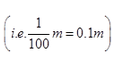wide and 80000 m long.

= 2(.01+80000) m = 2 x 80000.01 m = 160000.02 m

Offer running on EduRev: Apply code STAYHOME200 to get INR 200 off on our premium plan EduRev Infinity!

,

,

,

,

,

,

,

,

,

,

,

,

,

,

,

,

,

,

,

,

,

,

,

,

,

,

,

,

,

,

;